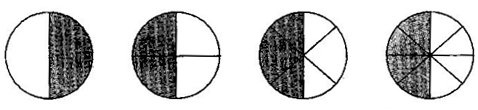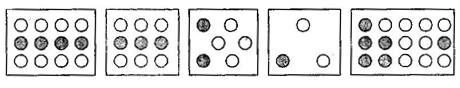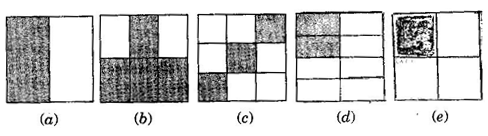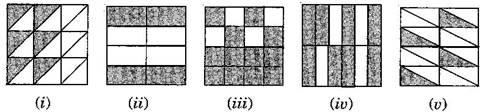1. /
2. CBSE
3. /
4. Class 06
5. /
6. Mathematics
7. /
8. NCERT Solutions for Class...

# NCERT Solutions for Class 6 Maths Exercise 7.3

NCERT solutions for Maths Fractions## NCERT Solutions for Class 6 Maths Fractions

###### Question 1.Write the fractions. Are all these fractions equivalent:

(a)(b)(a) {tex}\frac{1}{2},\frac{2}{4},\frac{3}{6},\frac{4}{8}{/tex}

Yes, all of these fractions are equivalent.

(b) {tex}\frac{4}{12},\frac{3}{9},\frac{2}{6},\frac{1}{3},\frac{6}{15}{/tex}

No, these fractions are not equivalent.

###### Question 2.Write the fraction and pair up the equivalent fractions to each row:(a){tex}\frac{1}{2}{/tex}

(ii) {tex}\frac{4}{8}=\frac{1}{2}{/tex}

(b) {tex}\frac{4}{6}=\frac{2}{3}{/tex}

(iv) {tex}\frac{8}{12}=\frac{2}{3}{/tex}

(c) {tex}\frac{3}{9}=\frac{1}{3}{/tex}

(i) {tex}\frac{6}{18}=\frac{1}{3}{/tex}

(d) {tex}\frac{2}{8}=\frac{1}{4}{/tex}

(v) {tex}\frac{4}{16}=\frac{1}{4}{/tex}

(e) {tex}\frac{3}{4}{/tex}

(iii) {tex}\frac{12}{16}=\frac{3}{4}{/tex}

NCERT Solutions for Class 6 Maths Exercise 7.3

###### Question 3.Replace {tex}\boxed{\,\,\,\,\,}{/tex} in each of the following by the correct number:

(a) {tex}\frac{2}{7} = \frac{8}{{\boxed{{\text{ }}}}}{/tex}

(b) {tex}\frac{5}{8} = \frac{{10}}{{\boxed{{\text{ }}}}}{/tex}

(c) {tex}\frac{3}{5} = \frac{{\boxed{{\text{ }}}}}{{20}}{/tex}

(d) {tex}\frac{{45}}{{60}} = \frac{{15}}{{\boxed{{\text{ }}}}}{/tex}

(e) {tex}\frac{{18}}{{24}} = \frac{{\boxed{{\text{ }}}}}{4}{/tex}

(a) {tex}\frac{2}{7} = \frac{{2 \times 4}}{{7 \times 4}} = \frac{8}{{\boxed{28}}}{/tex}

(b) {tex}\frac{5}{8} = \frac{{5 \times 2}}{{8 \times 2}} = \frac{{10}}{{\boxed{16}}}{/tex}

(c) {tex}\frac{3}{5} = \frac{{3 \times 4}}{{5 \times 4}} = \frac{{\boxed{12}}}{{20}}{/tex}

(d) {tex}\frac{{45}}{{60}} = \frac{{45 \div 3}}{{60 \div 3}} = \frac{{15}}{{\boxed{20}}}{/tex}

(e) {tex}\frac{{18}}{{24}} = \frac{{18 \div 6}}{{24 \div 6}} = \frac{{\boxed3}}{4}{/tex}

###### Question 4.Find the equivalent fraction of {tex}\frac{3}{5}{/tex} having:

(a) denominator 20

(b) numerator 9

(c) denominator 30

(d) numerator 27

(a) {tex}\frac{3}{5} = \frac{{3 \times 4}}{{5 \times 4}} = \frac{{12}}{{20}}{/tex}

(b) {tex}\frac{3}{5} = \frac{{3 \times 3}}{{5 \times 3}} = \frac{9}{{15}}{/tex}

(c) {tex}\frac{3}{5} = \frac{{3 \times 6}}{{5 \times 6}} = \frac{{18}}{{30}}{/tex}

(d) {tex}\frac{3}{5} = \frac{{3 \times 9}}{{5 \times 9}} = \frac{{27}}{{45}}{/tex}

NCERT Solutions for Class 6 Maths Exercise 7.3

###### Question 5.Find the equivalent fraction of {tex}\frac{{36}}{{48}}{/tex} with:

(a) numerator 9

(b) denominator 4

(a) {tex}\frac{{36}}{{48}} = \frac{{36 \div 4}}{{48 \div 4}} = \frac{9}{{12}}{/tex}

(b) {tex}\frac{{36}}{{48}} = \frac{{36 \div 12}}{{48 \div 12}} = \frac{3}{4}{/tex}

###### Question 6.Check whether the given fraction are equivalent:

(a) {tex}\frac{5}{9},\frac{{30}}{{54}}{/tex}

(b) {tex}\frac{3}{{10}},\frac{{12}}{{50}}{/tex}

(c){tex}\frac{7}{{13}},\frac{5}{{11}}{/tex}

(a) {tex}\frac{5}{9},\frac{{30}}{{54}}{/tex} = {tex}\frac{{5 \times 6}}{{9 \times 6}},\frac{{30}}{{54}}{/tex} = {tex}\frac{{30}}{{54}},\frac{{30}}{{54}}{/tex}

Therefore, {tex}\frac{5}{9},\frac{{30}}{{54}}{/tex} are equivalent.

(b) {tex}\frac{3}{{10}},\frac{{12}}{{50}}{/tex} = {tex}\frac{{3 \times 5}}{{10 \times 5}},\frac{{12}}{{50}}{/tex} = {tex}\frac{{15}}{{50}},\frac{{12}}{{50}}{/tex}

Therefore, {tex}\frac{3}{{10}},\frac{{12}}{{50}}{/tex} are not equivalent.

(c) {tex}\frac{7}{{13}},\frac{5}{{11}}{/tex} = {tex}\frac{{7 \times 11}}{{13 \times 11}},\frac{{5 \times 13}}{{11 \times 13}}{/tex} = {tex}\frac{{77}}{{143}},\frac{{65}}{{143}}{/tex}

Therefore, {tex}\frac{7}{{13}},\frac{5}{{11}}{/tex} are not equivalent fraction.

NCERT Solutions for Class 6 Maths Exercise 7.3

###### Question 7.Reduce the following fractions to simplest form:

(a) {tex}\frac{{48}}{{60}}{/tex}

(b) {tex}\frac{{150}}{{60}}{/tex}

(c) {tex}\frac{{84}}{{98}}{/tex}

(d) {tex}\frac{{12}}{{52}}{/tex}

(e) {tex}\frac{7}{{28}}{/tex}

(a) {tex}\frac{48}{60}=\frac{2\times 2\times 2\times 2\times 3}{2\times 2\times 3\times 5}=\frac{4}{5}{/tex}

(b) {tex}\frac{150}{60}=\frac{3\times 5\times 10}{2\times 3\times 10}=\frac{5}{2}{/tex}

(c) {tex}\frac{84}{98}=\frac{2\times 3\times 14}{7\times 14}=\frac{6}{7}{/tex}

(d) {tex}\frac{12}{52}=\frac{2\times 2\times 3}{2\times 2\times 13}=\frac{3}{13}{/tex}

(e) {tex}\frac{7}{28}=\frac{7}{2\times 2\times 7}=\frac{1}{4}{/tex}

###### Question 8.Ramesh had 20 pencils, Sheelu had 50 pencils and Jamaal had 80 pencils. After 4 months, Ramesh used up 10 pencils, Sheelu used up 25 pencils and Jamaal used up 40 pencils. What fraction did each use up? Check is each has used up an equal fraction of her/his pencils?

Ramesh: Total pencils = 20

Pencils used = 10

Fraction = {tex}\frac{10}{20}=\frac{1}{2}{/tex}

Sheelu: Total pencils = 50

Pencils used = 25

Fraction = {tex}\frac{25}{50}=\frac{1}{2}{/tex}

Jamaal: Total pencils = 80

Pencils used = 40

Fraction = {tex}\frac{40}{80}=\frac{1}{2}{/tex}

Since, all of them used half of their pencils, therefore each one used up equal fraction of pencils.

NCERT Solutions for Class 6 Maths Exercise 7.3

###### Question 9. Match the equivalent fractions and write two more for each:
 (i){tex}\frac{{250}}{{400}}{/tex} (a) {tex}\frac{2}{3}{/tex} (ii){tex}\frac{{180}}{{200}}{/tex} (b) {tex}\frac{2}{5}{/tex} (iii){tex}\frac{{660}}{{990}}{/tex} (c) {tex}\frac{1}{2}{/tex} (iv){tex}\frac{{180}}{{360}}{/tex} (d) {tex}\frac{5}{8}{/tex} (v){tex}\frac{{220}}{{550}}{/tex} (e) {tex}\frac{9}{{10}}{/tex}

(i) {tex}\frac{250}{400}=\frac{5}{8},\frac{10}{16},\frac{15}{24}{/tex}

(d) {tex}\frac{5}{8}{/tex}

(ii) {tex}\frac{180}{200}=\frac{9}{10},\frac{18}{20},\frac{27}{30}{/tex}

(e) {tex}\frac{9}{10}{/tex}

(iii) {tex}\frac{660}{990}=\frac{2}{3},\frac{4}{6},\frac{6}{9}{/tex}

(a) {tex}\frac{2}{3}{/tex}

(iv) {tex}\frac{180}{360}=\frac{1}{2},\frac{2}{4},\frac{3}{6}{/tex}

(c){tex}\frac{1}{2}{/tex}

1. {tex}\frac{220}{550}=\frac{2}{5},\frac{4}{10},\frac{6}{15}{/tex}

(b) {tex}\frac{2}{5}{/tex}

## NCERT Solutions for Class 6 Maths Exercise 7.3

NCERT Solutions Class 6 Maths PDF (Download) Free from myCBSEguide app and myCBSEguide website. Ncert solution class 6 Maths includes text book solutions from Class 6 Maths Book . NCERT Solutions for CBSE Class 6 Maths have total 14 chapters. 6 Maths NCERT Solutions in PDF for free Download on our website. Ncert Maths class 6 solutions PDF and Maths ncert class 6 PDF solutions with latest modifications and as per the latest CBSE syllabus are only available in myCBSEguide.

## CBSE app for Students

To download NCERT Solutions for Class 6 Maths, Social Science Computer Science, Home Science, Hindi English, Maths Science do check myCBSEguide app or website. myCBSEguide provides sample papers with solution, test papers for chapter-wise practice, NCERT solutions, NCERT Exemplar solutions, quick revision notes for ready reference, CBSE guess papers and CBSE important question papers. Sample Paper all are made available through the best app for CBSE students and myCBSEguide website.Test Generator

Create question paper PDF and online tests with your own name & logo in minutes.myCBSEguide

Question Bank, Mock Tests, Exam Papers, NCERT Solutions, Sample Papers, Notes

### 1 thought on “NCERT Solutions for Class 6 Maths Exercise 7.3”

1. Example correctL